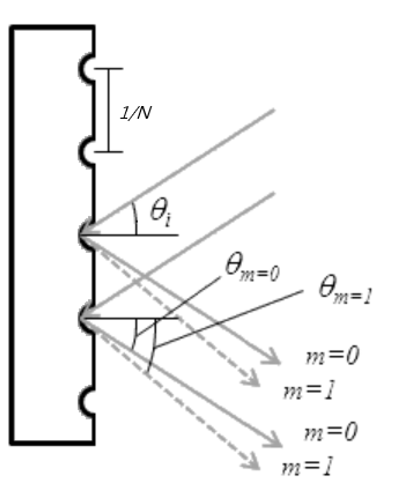# Diffracted Angle and Littrow Angle of Reflective Gratings

This Calculator Will Help You To calculate The Diffracted Angle For A Given Incidence Angle and The Littrow Angle of Reflective Gratings.If m is negative, it means that the diffracted light and diffracted light are on the same side of the normal. If m is positive, it means that the diffracted light and diffracted light are on the opposite side of the normal.

### $\theta$=arcsin$\frac{N m \lambda}{2}$

Raster Line Density $N$; Incidence Angle $\theta_i$; Order $m$; Wavelength $\lambda$
Diffracted Angle $\theta_m$; Littrow Angle $\theta$;

Input
1/mm
degree (°)

nm

Output
degree (°)

degree (°)